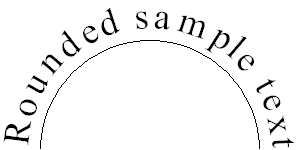This documentation is for the old version. Go to the latest Graphics Mill docs

# Measuring Characters Positions

To obtain each character position of a single-line text sting drawn by the GdiGraphics.DrawString method, use method Font.GetCharacterPositions(String, Single). It returns an array containing the X coordinate of each character in a given text string. If font tracking is modified with Tracking property, it is taken into account. The code example below demonstrates how to use this array to draw text on the curve:This image was produced with the following code:

Visual Basic
```Const centerX As Integer = 150
Const centerY As Integer = 150
Const radius As Integer = 110

'Create Bitmap object
Dim bitmap As New Aurigma.GraphicsMill.Bitmap(300, 150, _
Aurigma.GraphicsMill.PixelFormat.Format24bppRgb)

Dim graphics As Aurigma.GraphicsMill.Drawing.GdiGraphics = _
bitmap.GetGdiGraphics()

'Fill background
graphics.FillRectangle(New Aurigma.GraphicsMill.Drawing.SolidBrush( _
Aurigma.GraphicsMill.RgbColor.White), 0, 0, bitmap.Width, bitmap.Height)

Dim font As New Aurigma.GraphicsMill.Drawing.Font( _
"Times New Roman", 37, False, False)
font.VerticalAlignment = Aurigma.GraphicsMill.Drawing.VerticalAlignment.Bottom
font.HorizontalAlignment = Aurigma.GraphicsMill.Drawing.HorizontalAlignment.Center

Dim text As String = "Rounded sample text"

'Get characters positions
Dim positions As Single() = font.GetCharacterPositions(text, 0)
'Get string width
Dim strWidth As Single = font.MeasureString(text).Width
'Get string height
Dim strHeight As Single = font.MeasureString(text).Height

'Draw ellipse
graphics.DrawEllipse(New Aurigma.GraphicsMill.Drawing.Pen( _
Aurigma.GraphicsMill.RgbColor.Black, 1), 40, 40, 220, 220)

Dim brush As New Aurigma.GraphicsMill.Drawing.SolidBrush(Aurigma.GraphicsMill.RgbColor.Black)

'Draw character by character
For i As Integer = 0 To text.Length - 1
Dim charWidth As Single = font.GetBlackBox(text(i)).Width

'Compute angle to which current character will be rotated. Rotation will be
'performed relative to the middle of the bottom side of the character.
Dim angle As Single = Math.PI - (positions(i) + charWidth / 2.0F) * Math.PI / strWidth

'Compute the x and y coordinate of the bottom/top* middle* of the character
Dim x As Single = centerX + radius * Math.Cos(angle)
Dim y As Single = centerY - radius * Math.Sin(angle)

Dim rotateMatrix As New System.Drawing.Drawing2D.Matrix()

'Convert angle from radians to degrees
Dim degAngle As Single = angle / Math.PI * 180
rotateMatrix.RotateAt(90 - degAngle, New System.Drawing.PointF(x, y))
graphics.Transform = rotateMatrix
graphics.DrawString(text(i).ToString(), font, brush, x, y)
Next
```
C#
```const int centerX = 150;
const int centerY = 150;

//Create Bitmap object
Aurigma.GraphicsMill.Bitmap bitmap =
new Aurigma.GraphicsMill.Bitmap(300, 150, Aurigma.GraphicsMill.PixelFormat.Format24bppRgb);

Aurigma.GraphicsMill.Drawing.GdiGraphics graphics = bitmap.GetGdiGraphics();

//Fill background
graphics.FillRectangle(new Aurigma.GraphicsMill.Drawing.SolidBrush(
Aurigma.GraphicsMill.RgbColor.White), 0, 0, bitmap.Width, bitmap.Height);

Aurigma.GraphicsMill.Drawing.Font font =
new Aurigma.GraphicsMill.Drawing.Font("Times New Roman", 37, false, false);
font.VerticalAlignment = Aurigma.GraphicsMill.Drawing.VerticalAlignment.Bottom;
font.HorizontalAlignment = Aurigma.GraphicsMill.Drawing.HorizontalAlignment.Center;

string text = "Rounded sample text";

//Get characters positions
float[] positions = font.GetCharacterPositions(text, 0);
//Get string width
float strWidth = font.MeasureString(text).Width;
//Get string height
float strHeight = font.MeasureString(text).Height;

//Draw ellipse
graphics.DrawEllipse(new Aurigma.GraphicsMill.Drawing.Pen(
Aurigma.GraphicsMill.RgbColor.Black, 1), 40, 40, 220, 220);

Aurigma.GraphicsMill.Drawing.SolidBrush brush =
new Aurigma.GraphicsMill.Drawing.SolidBrush(Aurigma.GraphicsMill.RgbColor.Black);

//Draw character by character
for (int i = 0; i < text.Length; i++)
{
float charWidth = font.GetBlackBox(text[i]).Width;

//Compute angle to which rotate current character. Rotation will be
//performed relative to the middle of the bottom side of the character.
float angle = (float)(Math.PI - (positions[i] + charWidth / 2.0f) * Math.PI / strWidth);

//Compute the x and y coordinate of the bottom/top* middle* of the character
float x = (float)(centerX + radius * Math.Cos(angle));
float y = (float)(centerY - radius * Math.Sin(angle));

System.Drawing.Drawing2D.Matrix rotateMatrix = new System.Drawing.Drawing2D.Matrix();

//Convert angle from radians to degrees
float degAngle = (float)(angle / Math.PI * 180);

rotateMatrix.RotateAt(90 - degAngle, new System.Drawing.PointF(x, y));

graphics.Transform = rotateMatrix;

graphics.DrawString(text[i].ToString(), font, brush, x, y);
}
```